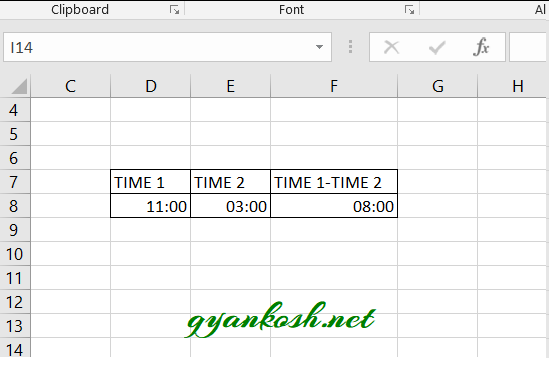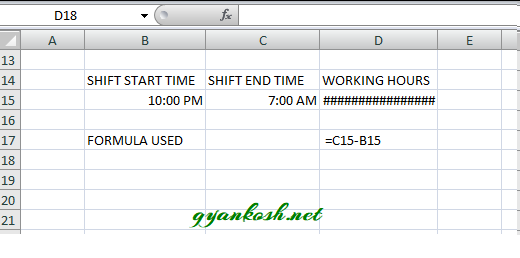PROVIDING ACCURATE, RELIABLE AND STRAIGHT TO THE POINT KNOWLEDGE ABOUT DIFFERENT IT TOOLS EXPLAINED WITH PRACTICAL EXAMPLES

# HOW TO SUBTRACT TIME IN EXCEL

## INTRODUCTION

DATES and TIME , if you are new to Excel and you have already tried using those format, I am pretty sure that you must have felt panicky at some sort of time.
It is quite obvious because when we don’t know the exact working of any system or process, we try to use it just by hit and trial method. It works sometimes and sometimes not.
Here we are talking about Dates and Time in Excel. These are the tricky formats which we need frequently in our reports or charts.
Many times, we need to do operations on them. We need to put them in the conditions to trigger some event which makes it very important for us to learn the exact procedures to perform a task concerned with the dates and time.
In this article we would learn different tricks and methods to handle and manipulate Dates and Time formats so that they don’t mess up with our reports.

One of such requirement is to subtract time in Excel.
BEFORE READING THIS ARTICLE , IT IS REQUESTED TO VISIT THE PART I WHICH DISCUSSES THE CONCEPT OF THE DATE AND TIME IN EXCEL FOR BETTER UNDERSTANDING.CLICK HERE TO VISIT.
In this article we would learn various ways to subtract the time correctly in Excel.

## SUBTRACT TIME IN EXCEL

Let us try to learn about how to subtract time in Excel.

### CONCEPT:

When we are creating any worksheet regarding the time, addition and subtraction are the common operations which have to come in our way. So, it is very important to be very clear about how the time work when it is subtracted.
In this section, let us try to learn how we can subtract the time in excel.

Time is just another number for Excel. We can subtract it the same way as numbers but with a bit care.

Just like the addition, we can simply subtract the time arithmetically. For example

If a cell contains 12:00 and one contains 4:00 we can simply subtract the 12:00-4:00 and the result would come as 8:00The formula used is D8-E8.

Let us try the different cases and learn how to tackle different situations.

### EXAMPLE 1: SIMPLE DIFFERENCE BETWEEN TIMES.

TIME 1: 15:00 HRS

TIME 2: 8:00 HRS

DIFFERENCE= TIME 1- TIME 2= 7:00

As shown in the picture below.

### EXAMPLE 2: SUBTRACTING THE TIME DURING NIGHT HOURS

#### CONCEPT:

This particular case is for certain situations where we are calculating the work hours for a night shift.

Mostly, the night shift starts on the day 1 and ends in the morning of day 2.

This is the situation where our simple subtraction fails.

Let us try an example where the night shift starts at 10 pm and ends at 7 am.

Let us try to find out the work hours.We put the formula as SHIFT END TIME-SHIFT START TIME [C15-B15 FOR OUR EXAMPLE] but the result didn’t show and #### appeared.

It appears when the result is negative.

WHEN WE DOESN’T MENTION THE DATE WITH A TIME, THE DEFAULT DATE IS TAKEN WHICH IS JAN 1 , 1900. ThE TIME DOESN’T GO WITHOUT A DATE WHETHER YOU MENTION IT OR NOT.

Let us try to sort this problem out.

#### STEPS TO FIND OUT THE TIME DIFFERENCE INCLUDING NIGHT HOURS [NIGHT SHIFT CASE]

• Put the TIME 1 as SHIFT START TIME, which is 10:00 PM in our example.
• Put the TIME 2 as SHIFT END TIME , which is 7:00 AM in our example.
• In the Working hours put the formula as
•  =TEXT(IF(C15<B15,C15+1-B15,C15-B15),”[HH]:MM”)
• The formula adds a day to the morning time to make it the time of the next day so that we can perform our calculations.
• Text function is used to make the format to count hours only.
• If we need to calculate the time including many days, we need to enter dates too with the time.
• We can simply subtract the time if dates are incorporated.

### EXAMPLE 3: SUBTRACTING THE TIME WHEN DATE IS ALSO MENTIONED

#### CONCEPT:

This particular case is for certain situations where we are calculating the work hours day or night for the different dates. Example 2 has been discussed specifically for the NIGHT SHIFTS only.

This example discusses the tasks which starts on a particular date any time and ends up after a few days at some given time.
For example suppose the Brick Work of a room started at 4 pm 10.08.2020 and ended on 2 pm 15.08.2020.
Let us find out the total duration in hours for this data.

It appears when the result is negative.

THE DATE AND TIME CAN BE ADDED TOGETHER TO CREATE THE COMPLETE NUMBER INCORPORATING THE DATE AND TIME TOGETHER. IT CAN BE SIMPLY SUBTRACTED AND CONVERTED BACK TO THE FORMAT WE WANT.

Let us try to sort this problem out.

#### STEPS TO FIND OUT THE TIME DIFFERENCE BETWEEN TWO TIME ON DIFFERENT DATES

• Put the Date and Time in the respective cells for the BRICKWORK START TIME [ CELL G7]  as 10-08-2020 4 pm.
• Put the Date and Time in the respective cells for the BRICKWORK END TIME [ CELL G8 ]as 15-08-2020 2 pm
• In the TOTAL BRICK WORK TIME CELL G12 put the formula as
• =TEXT((G8+H8)-(G7+H7),”[HH]:MM”)
• The formula adds the date and time together for creating a complete time so that we can perform our calculations.
• Text function is used to make the format to count hours only.
• We can simply subtract the time if dates are incorporated.

• ## WHAT IS EXCEL, HOW EXCEL WORKS, WRITE FORMULA IN EXCEL, EXAMPLE OF USING FORMULA IN EXCEL, ANIMATED EXAMLPE OF USING FORMULA IN EXCEL

LEARN

• EXCEL
• JAVASCRIPT
• MORE TO COME…

Encourage us by following at …

If you have any queries or suggestions or any problem which you would like us to solve for you, kindly mail at

[email protected]